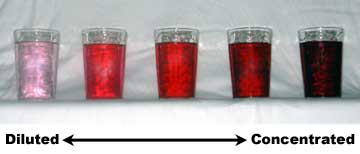## Dilutions of Solutions

#### Learning Objective

• Calculate the concentration of a diluted solution.

#### Key Points

• Most commonly, a solution’s concentration is expressed in terms of mass percent, mole fraction, molarity, molality, and normality. When calculating dilution factors, it is important that the units of volume and concentration remain consistent.
• Dilution calculations can be performed using the formula M1V1 = M2V2.
• A serial dilution is a series of stepwise dilutions, where the dilution factor is held constant at each step.

#### Terms

• dilutiona solution that has had additional solvent, such as water, added to make it less concentrated
• serial dilutionstepwise dilution of a substance in solution

Dilution refers to the process of adding additional solvent to a solution to decrease its concentration. This process keeps the amount of solute constant, but increases the total amount of solution, thereby decreasing its final concentration. Dilution can also be achieved by mixing a solution of higher concentration with an identical solution of lesser concentration. Diluting solutions is a necessary process in the laboratory, as stock solutions are often purchased and stored in very concentrated forms. For the solutions to be usable in the lab (for a titration, for instance), they must be accurately diluted to a known, lesser concentration.

The volume of solvent needed to prepare the desired concentration of a new, diluted solution can be calculated mathematically. The relationship is as follows:

$M_1V_1=M_2V_2$

M1 denotes the concentration of the original solution, and V1 denotes the volume of the original solution; M2 represents the concentration of the diluted solution, and V2 represents the final volume of the diluted solution. When calculating dilution factors, it is important that the units for both volume and concentration are the same for both sides of the equation.

## Example

• 175 mL of a 1.6 M aqueous solution of LiCl is diluted with water to a final volume of 1.0 L. What is the final concentration of the diluted solution?
• $M_1V_1=M_2V_2$
• (1.6 M)(175 mL) = M2(1000 mL)
• M2 = 0.28 MDilutionsDilutions can sometimes be visually observed. In the image above, the intense red color slowly fades as the solutions become more diluted.

## Serial Dilutions

Serial dilutions involve diluting a stock or standard solution multiple times in a row. Typically, the dilution factor remains constant for each dilution, resulting in an exponential decrease in concentration. For example, a ten-fold serial dilution could result in the following concentrations: 1 M, 0.1 M, 0.01 M, 0.001 M, and so on. As is evidenced in this example, the concentration is reduced by a factor of ten in each step. Serial dilutions are used to accurately create extremely diluted solutions, as well as solutions for experiments that require a concentration curve with an exponential or logarithmic scale. Serial dilutions are widely used in experimental sciences, including biochemistry, pharmacology, microbiology, and physics.